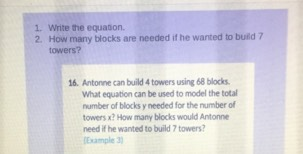### ¿Todavía tienes preguntas de matemáticas?

Pregunte a nuestros tutores expertos
Algebra
Pregunta1. Write the equation.

2. How many blocks are needed if he wanted to build $$7$$ towers?

16. Antonne can build $$4$$ towers using $$68$$ blocks. What equation can be used to model the total number of blocks y needed for the number of towers $$x$$ ? How many blocks would Antonne need if he wanted to build $$7$$ towers? (Example 3)